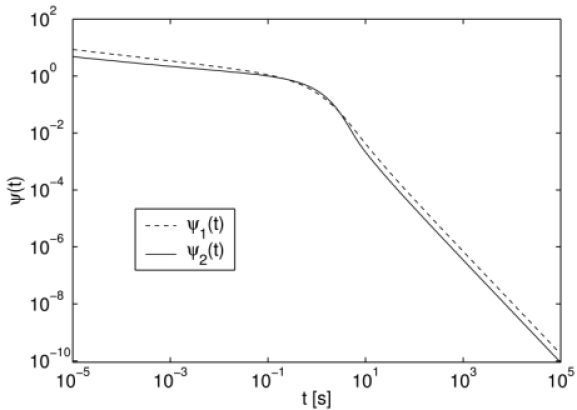Sie sind hier: ICP » R. Hilfer » Publikationen

# 2 Definition of Models

[2.3.1] Consider first the integral equation of motion for the CTRW-model [9, 10]. [2.3.2] The probability density pr,t obeys the integral equation

 p⁢r,t=δr⁢0⁢Φ⁢t+∫0tψ⁢t-t′⁢∑r′λ⁢r-r′⁢p⁢r′,t′⁢d⁢t′ (3)

where λr denotes the probability for a displacement r in each single step, and ψt is the waiting time distribution giving the probability density for the time interval t between two consecutive steps. [2.3.3] The transition probabilities obey rλr=1, and the function Φt is the survival probability at the initial position which is related to the waiting time distribution through

 Φ⁢t=1-∫0tψ⁢t′⁢d⁢t′. (4)

[page 3, §0]   Fourier-Laplace transformation leads to the solution in Fourier-Laplace space given as 

 p⁢k,u=1u⁢1-ψ⁢u1-ψ⁢u⁢λ⁢k (5)

where pk,u is the Fourier-Laplace transform of pr,t and similarly for ψ and λ.

[3.1.1] Two lattice models with different waiting time density will be considered. [3.1.2] In the first model the waiting time density is chosen as the one found in [1, 2]

 ψ1⁢t=tα-1τα⁢Eα,α⁢-tατα, (6)

where 0<α1,0<τ< is the characteristic time, and

 Ea,b⁢x=∑k=0∞xkΓ⁢a⁢k+b⁢a>0,b∈C. (7)

is the generalized Mittag-Leffler function . [3.1.3] In the second model the waiting time density is chosen as

 ψ2⁢t=tα-12⁢c⁢τ2⁢Eα,α⁢-tαc⁢τ2+12⁢τ⁢exp⁡-t/τ (8)

where 0<α1, 0<τ< and c>0 is a suitable dimensional constant.

[3.2.1] The waiting time density ψ2t differs only little from ψ1t as shown graphically in Figure 1.Figure 1: Waiting time densities ψ1t for model 1 and ψ2t for model 2 with α=0.8, τ=1 s and c=1 s-1.2.

[3.2.2] Note that both models have a long time tail of the form given in eq. (2), and the average waiting time 0tψitdt diverges.

[3.3.1] For both models the spatial transition probabilities are chosen as those for nearest-neighbour transitions (Polya walk) on a d-dimensional hypercubic lattice given as

 λ⁢r=12⁢d⁢∑j=1dδr,-σ⁢ej+δr,σ⁢ej (9)

where ej is the j-th unit basis vector generating the lattice, σ>0 is the lattice constant and δr,s=1 for r=s and δr,s=0 for rs.